## How to Convert Square Miles to Square Kilometers | Area Units

The image below represents square miles to square kilometers.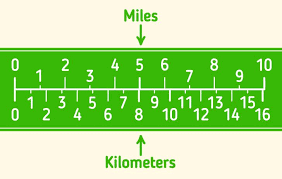To convert square miles to square kilometers, one essential parameter is needed and this parameter is Unit Value.

The formula for converting square miles to square kilometers:

1 Square Mile = 2.589988 Square Kilometers

Let’s solve an example;
Find the conversion of square miles to square kilometers when the unit value is 8.

1 Square Mile = 2.589988 Square Kilometers

Then,

8 Square Miles = (8 x 2.589988) Square Kilometers
8 Square Miles = 20.719904 Square Kilometers

Therefore, the square kilometer(s) is 20.719.

## How to Convert Acres to Kilometers | Area Units

The image below represents acres to square kilometers.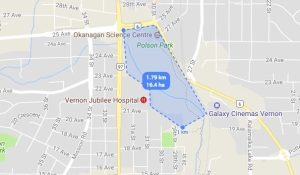To convert acres to square kilometers, one essential parameter is needed and this parameter is Unit Value.

The formula for converting acres to square kilometers:

1 Acre = 0.004047 Square Kilometers

Let’s solve an example;
Find the conversion of acres to square kilometers when the unit value is 7.

1 Acre = 0.004047 Square Kilometers

Then,

7 Acres = (7 x 0.004047) Square Kilometers
7 Acres = 0.028329 Square Kilometers

Therefore, the square kilometer(s) is 0.028329.

## How to Convert Square Yards to Square Kilometers | Area Units

The image below represents square yards to square kilometers.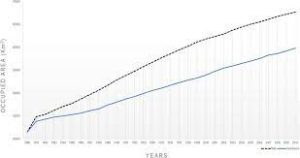To convert square yards to square kilometers, one essential parameter is needed and this parameter is Unit Value.

The formula for converting square yards to square kilometers:

1 Square Yard = 0.00000083612736 Square Kilometers

Let’s solve an example;
Find the conversion of square yards to square kilometers when the unit value is 14.

1 Square Yard = 0.00000083612736 Square Kilometers

Then,

14 Square Yards = (14 x 0.00000083612736) Square Kilometers
14 Square Yards = 0.00001170578304 Square Kilometers

Therefore, the square kilometer(s) is 0.0000117.

## How to Convert Square Feet to Square Kilometers | Area Units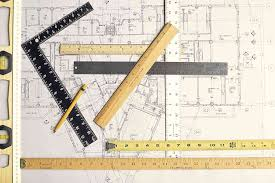The image above represents square feet to square kilometers.

To convert square feet to square kilometers, one essential parameter is needed and this parameter is Unit Value.

The formula for converting square feet to square kilometers:

1 Square Feet = 0.00000009290304 Square Kilometers

Let’s solve an example;
Find the conversion of square feet to square kilometers when the unit value is 13.

1 Square Feet = 0.00000009290304 Square Kilometers

Then,

13 Square Feet = (13 x 0.00000009290304) Square Kilometers
13 Square Feet = 0.0000012077395199999999 Square Kilometers

Therefore, the square kilometer(s) is 0.000001207.

## How to Convert Square Inches to Square Kilometers | Area Units

The image below represents square inches to square kilometers.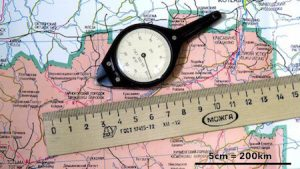To convert square inches to square kilometers, one essential parameter is needed and this parameter is Unit Value.

The formula for converting square inches to square kilometers:

1 Square Inch = 0.00000000064516 Square Kilometers

Given an example;
Convert square inches to square kilometers when the unit value is 7.

1 Square Inch = 0.00000000064516 Square Kilometers

Then,

7 Square Inches = (7 x 0.00000000064516) Square Kilometers
7 Square Inches = 4.51612e-9 Square Kilometers

Therefore, the square kilometer(s) is 4.51612e-9.

## How to Convert Square Kilometers to Square Miles | Area Units

The image below represents square kilometers to square miles.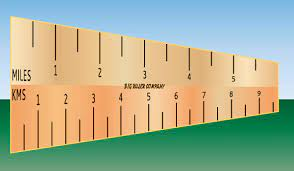The conversion of square kilometers to square miles requires one essential parameter and this parameter is Unit Value.

The formula for converting square kilometers to square miles:

1 Square Kilometer = 0.386102 Square Mile(s)

Let’s solve an example;
Find the conversion of square kilometers to square miles when the unit value is 14.

1 Square Kilometer = 0.386102 Square Mile(s)

Then,

14 Square Kilometers = (14 x 0.386102) Square Miles
14 Square Kilometers = 5.405428 Square Miles

Therefore, the square mile(s) is 5.405

## How to Convert Square Kilometers to Acres | Area Units

The image below represents square kilometers to acres.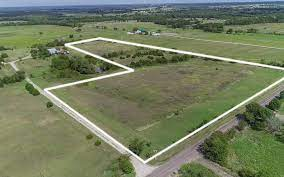The conversion of square kilometers to acres requires one essential parameter and this parameter is Unit Value.

The formula for converting square kilometers to acres:

1 Square Kilometer = 247.1054 Acres

Let’s solve an example;
Find the conversion of square kilometers to acres when the unit value is 2.

1 Square Kilometer = 247.1054 Acres

Then,

2 Square Kilometers = (2 x 247.1054) Acres
2 Square Kilometers = 494.2108 Acres

Therefore, the acre(s) is 494.21

## How to Convert Square Kilometers to Square Yards | Area Units

The image below represents square kilometers to square yards.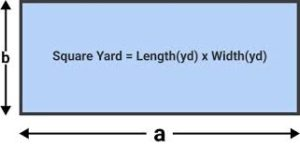The conversion of square kilometers to square yards requires one essential parameter and this parameter is Unit Value.

The formula for converting square kilometers to square yards:

1 Square Kilometer = 1195990 Square Yards

Given an example;
Find the conversion of square kilometers to square yards when the unit value is 22.

1 Square Kilometer = 1195990 Square Yards

Then,

22 Square Kilometers = (22 x 1195990) Square Yards
22 Square Kilometers = 26311780 Square Yards

Therefore, the square yard(s) is 26311780.

## How to Convert Square Kilometers to Square Feet | Area units

The image below represents square kilometers to square feet.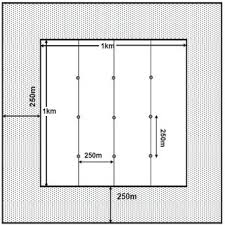The conversion of square kilometers to square feet requires one essential parameter and this parameter is Unit Value.

The formula for converting square kilometers to square feet:

1 Square Kilometer = 10763910 Square Feet

Let’s solve an example;
Given the unit value of 28. Find the conversion of square kilometers to square feet?

1 Square Kilometer = 10763910 Square Feet

Then,

28 Square Kilometers = (28 x 10763910) Square Feet
28 Square Kilometers = 301389480 Square Feet

Therefore, the square feet is 301389480.

## How to Convert Square Kilometers to Square Inches | Area Units

The image below represents square kilometers to square inches.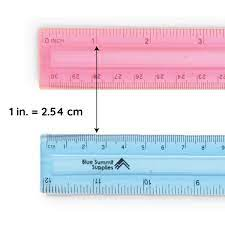The conversion of square kilometers to square inches requires one essential parameter and this parameter is Unit Value.

The formula for converting square kilometers to square inches:

1 Square Kilometer = 1550003100 Square Inches

Let’s solve an example;
Find the conversion of square kilometers to square inches when the unit value is 34.

1 Square Kilometer = 1550003100 Square Inches

Then,

34 Square Kilometers = (34 x 1550003100) Square Inches
34 Square Kilometers = 52700105400 Square Inches

Therefore, the square inch(es) is 52700105400.